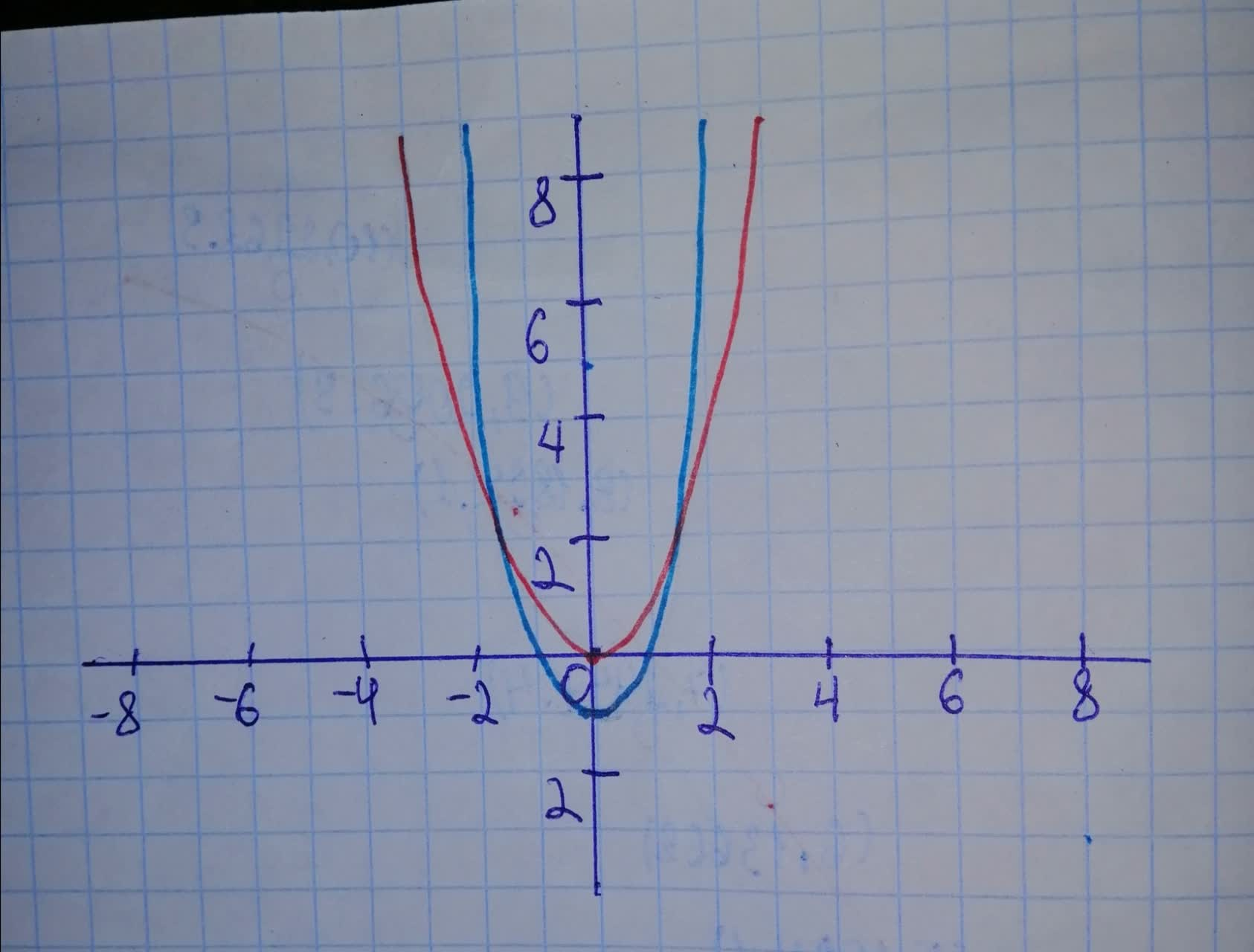# Sketch a graph of the function. Use transformations of functions whenever possible. f(x)=2x^{2} - 1Marvin Mccormick 2021-01-22 Answered
Sketch a graph of the function. Use transformations of functions whenever possible.
You can still ask an expert for help

• Questions are typically answered in as fast as 30 minutes

Solve your problem for the price of one coffee

• Math expert for every subject
• Pay only if we can solve itMalena
First graph function $y={x}^{2}$ Then, to obtain graph of function do following transformations: -stretch vertically by a factor of 2 - shift 1 unit downward On graph: Red - $y={x}^{2}$ Blue -